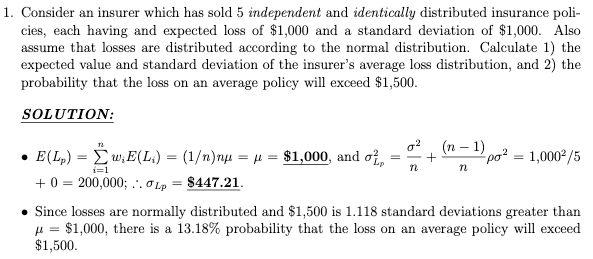# Risk Pooling Class Problem and plans for tomorrow’s meeting of Finance 4335

Here is the solution for the first question in the class problem that we worked on yesterday.Please check your work against this solution, and also solve problems 2 and 3.

In problem 2, we change the composition of the risk pool by adding an additional 5 risks.  While this change does not affect the average loss per policy (see equation 1a on page 4 of the lecture outline),  it lowers the average risk per policy (equation 2a on the same page).  The lowering of average risks causes the tail probability (i.e., the probability of an average loss exceeding \$1,500) to decline, which you will numerically confirm when you complete your work on problem 2.

In problem 3, we use the same number of risks (n = 10) as in problem 2, but introduce positive correlation ($\rho = .1$). This causes the average risk per policy to increase vis-a-vis the result shown in problem 2, which causes the tail probability to increase, which you will numerically confirm when  you complete your work on problem 3.

We’ll begin class tomorrow by finishing off the solutions to this risk pooling class problem and covering the following set of topics which appear on pp. 6-21 of the lecture outline @ http://fin4335.garven.com/fall2019/lecture5.pdf: (1) Making decisions under certainty, 2) Making decisions under uncertainty, 3) The expected value model, 4) The St. Petersburg Paradox, and 5) The expected utility model.